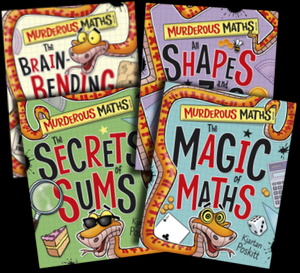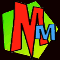The Magic of Maths

Some extra details to go with the Murderous Maths magic bookThink of a Number: The Magic 3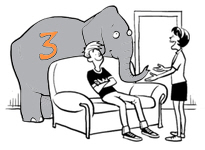How does it work?

This table shows the trick using the number 7, then we'll explain it using algebra. Instead of a number, we use letter x!

Letter X can represent ANY number. In the end all the x's disappear, which shows that it doesn't matter which number you choose to start with.

 Instructions: We'll "think" of number 7 Now we'll use x. Think of a number 7 x Add 2 9 x + 2 Multiply by 5 45 5(x+2) = 5x +10 Add 3 48 5x + 13 Multiply by 2 96 10x + 26 Add 4 100 10x + 30 Divide by 10 10 x + 3 Take away the number you first thought of and the answer will always be 3! 10 - 7 =3 x + 3 - x = 3

Think of a Number: Think of TWO numbers!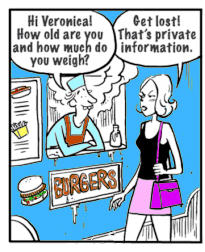Pongo asks Veronica to put her age and weight secretly into a calculator.

Later she is allowed to "take them" away so he can't know what they are.

Or can he...?

How does it work?

This trick involves some more complicated algebra. It uses two numbers - Veronica's age and her weight. We'll call these numbers A and B. In fact the trick can work with almost ANY two numbers, so long as number A is less than 100.
 This is what Pongo told Veronica to do: Age = 17 Weight = 55 Age = A Weight = B Put 13 into the calculator 13 13 Multiply by weight 13 x 55 = 715 13B Subtract 13 702 13B-13 Multiply by 7 4914 91B-91 Subtract 10 4904 91B - 101 Add the age 4904 + 17 = 4921 91B + A - 101 Multiply by 11 54131 1001B + 11A - 1111 Take away the age and take away the weight 54131 -17 -55 =54059 1000B + 10A -1111 Add 101 then show the answer! 54160 1000B + 10A -1010 To find the age and weight, take the zero off the answer (i.e. divide by 10) 5416 100B + A - 101 =100(B-1) + (A-1) To find A , add 1 to the last two digits 16 + 1 = 17 (A-1) + 1 = A To find B, add 1 to the first digits 54 + 1 = 55 (B-1)* + 1 = B * when the last two digits are removed, the 100(B-1) gets divided by 100 leaving just (B-1)

The Predictor Cards

Click the picture to see how to do the trick, or click the little picture for a sheet you can print out to make the cards!

The Seven Cards of Devious Trickery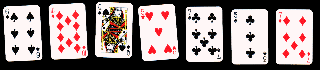Click the cards to see how Riverboat Lil does this same trick, and find out how it works!

The Fabulous Flexagons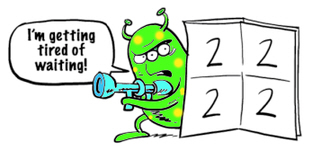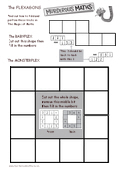Click the picture to find a template you can print out to make the BABYFLEX and the MONSTERFLEX.

You can see how to make some other flexagons at How to Make a Triflexagon

The Magic Ball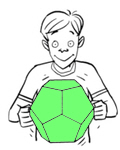Click the picture to find a template you can print out to make the DODECAHEDRON.

You can find more instructions at our dodecahedron Page

EXTRA TRICKS!

Try our Three Magical Colour Illusions!

Have you seen our Tricks and Games page?

You'll also find a few more tricks and things to do on our Teacher PageLINKS: